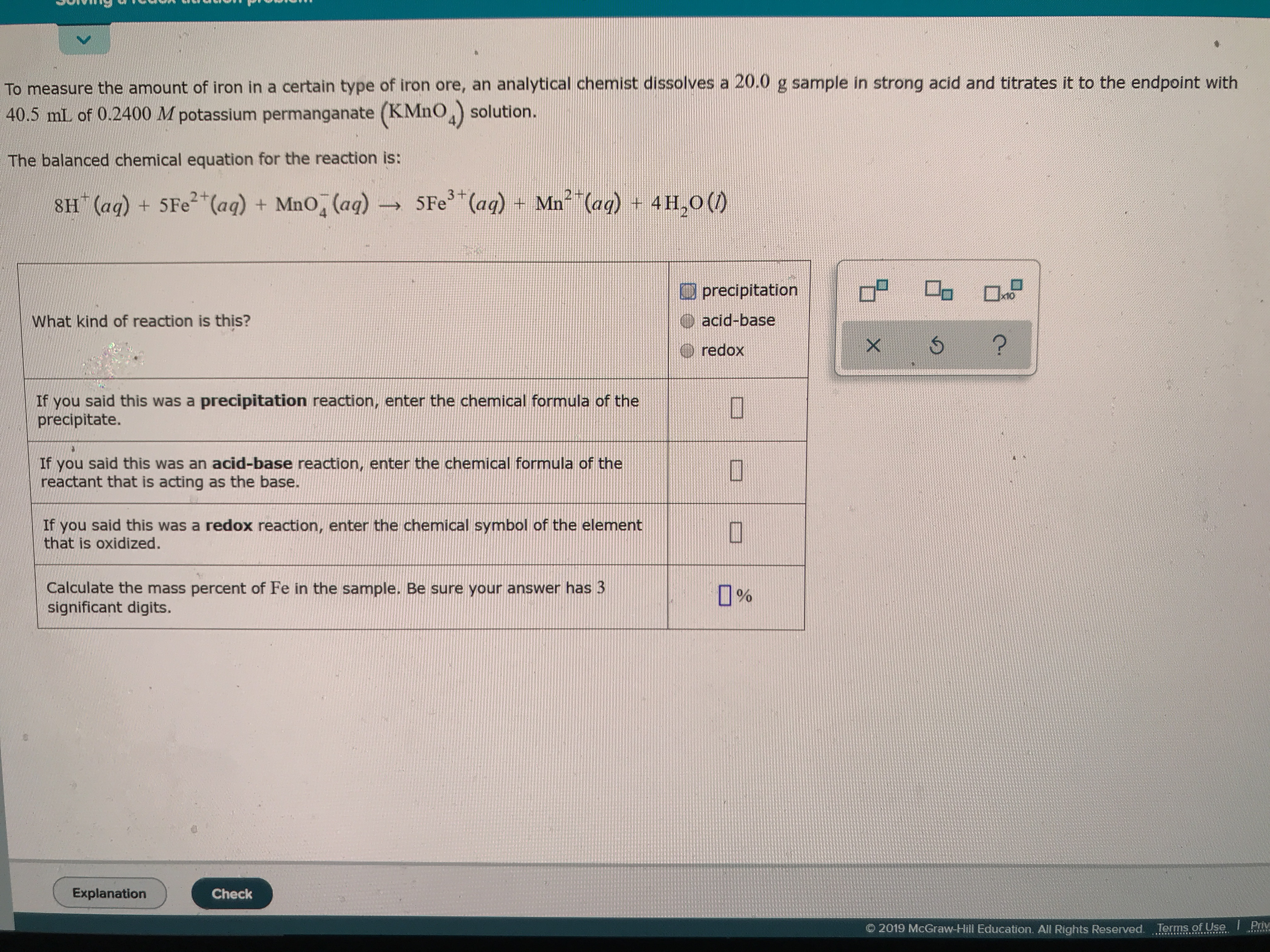# To measure the amount of iron in a certain type of iron ore, an analytical chemist dissolves a 20.0 g sample in strong acid and titrates it to the endpoint with 40.5 mL of 0.2400 M potassium permanganate (KMnO,) solution. The balanced chemical equation for the reaction is: 3 + 2+ 5Fe (aq) + Mn (aq) + 4 H,0 () 8H (aq) + 5Fe(aq) + MnO, (aq) precipitation acid-base What kind of reaction is this? ? redox If you said this was a precipitation reaction, enter the chemical formula of the precipitate. If you said this was an acid-base reaction, enter the chemical formula of the reactant that is acting as the base. said this was a redox reaction, enter the chemical symbol of the element If you that is oxidized. Calculate the mass percent of Fe in the sample. Be sure your answer has 3 significant digits 0% Explanation Check Priv 2019 McGraw-Hill Education. All Rights Reserved. Terms of Use

Question

What kind of reaction is this if it is. Precipitate write down the formula if you said acid-base right down the formula you said redox write down the formula then calculate the mass percent of FE in the sample be sure your answer has to be significant digits.help_outlineImage TranscriptioncloseTo measure the amount of iron in a certain type of iron ore, an analytical chemist dissolves a 20.0 g sample in strong acid and titrates it to the endpoint with 40.5 mL of 0.2400 M potassium permanganate (KMnO,) solution. The balanced chemical equation for the reaction is: 3 + 2+ 5Fe (aq) + Mn (aq) + 4 H,0 () 8H (aq) + 5Fe(aq) + MnO, (aq) precipitation acid-base What kind of reaction is this? ? redox If you said this was a precipitation reaction, enter the chemical formula of the precipitate. If you said this was an acid-base reaction, enter the chemical formula of the reactant that is acting as the base. said this was a redox reaction, enter the chemical symbol of the element If you that is oxidized. Calculate the mass percent of Fe in the sample. Be sure your answer has 3 significant digits 0% Explanation Check Priv 2019 McGraw-Hill Education. All Rights Reserved. Terms of Use fullscreen

### Want to see this answer and more?

Experts are waiting 24/7 to provide step-by-step solutions in as fast as 30 minutes!*

*Response times vary by subject and question complexity. Median response time is 34 minutes and may be longer for new subjects.
Tagged in
ScienceChemistry